### 3.5.9 Fixed Point Types

(1)
A fixed point type is either an ordinary fixed point type, or a decimal fixed point type. The error bound of a fixed point type is specified as an absolute value, called the delta of the fixed point type.
##### Syntax
```(2)
fixed_point_definition ::= ordinary_fixed_point_definition | decimal_fixed_point_definition
(3)
ordinary_fixed_point_definition ::=
delta static_expression  real_range_specification
(4)
decimal_fixed_point_definition ::=
delta static_expression digits static_expression [real_range_specification]
(5)
digits_constraint ::=
digits static_expression [range_constraint]
```
##### Name Resolution Rules
(6)
For a type defined by a fixed_point_definition, the delta of the type is specified by the value of the expression given after the reserved word delta; this expression is expected to be of any real type. For a type defined by a decimal_fixed_point_definition (a decimal fixed point type), the number of significant decimal digits for its first subtype (the digits of the first subtype) is specified by the expression given after the reserved word digits; this expression is expected to be of any integer type.
##### Legality Rules
(7)
In a fixed_point_definition or digits_constraint, the expressions given after the reserved words delta and digits shall be static; their values shall be positive.
(8)
The set of values of a fixed point type comprise the integral multiples of a number called the small of the type. For a type defined by an ordinary_fixed_point_definition (an ordinary fixed point type), the small may be specified by an attribute_definition_clause (see 13.3); if so specified, it shall be no greater than the delta of the type. If not specified, the small of an ordinary fixed point type is an implementation-defined power of two less than or equal to the delta.
(9)
For a decimal fixed point type, the small equals the delta; the delta shall be a power of 10. If a real_range_specification is given, both bounds of the range shall be in the range -(10**digits-1)*delta .. +(10**digits-1)*delta.
(10)
A fixed_point_definition is illegal if the implementation does not support a fixed point type with the given small and specified range or digits.
(11)
For a subtype_indication with a digits_constraint, the subtype_mark shall denote a decimal fixed point subtype.
##### Static Semantics
(12)
The base range (see 3.5) of a fixed point type is symmetric around zero, except possibly for an extra negative value in some implementations.
(13)
An ordinary_fixed_point_definition defines an ordinary fixed point type whose base range includes at least all multiples of small that are between the bounds specified in the real_range_specification. The base range of the type does not necessarily include the specified bounds themselves. An ordinary_fixed_point_definition also defines a constrained first subtype of the type, with each bound of its range given by the closer to zero of:
(14)
• the value of the conversion to the fixed point type of the corresponding expression of the real_range_specification;
(15)
• the corresponding bound of the base range.
(16)
A decimal_fixed_point_definition defines a decimal fixed point type whose base range includes at least the range -(10**digits-1)*delta .. +(10**digits-1)*delta. A decimal_fixed_point_definition also defines a constrained first subtype of the type. If a real_range_specification is given, the bounds of the first subtype are given by a conversion of the values of the expressions of the real_range_specification. Otherwise, the range of the first subtype is -(10**digits-1)*delta .. +(10**digits-1)*delta.
##### Dynamic Semantics
(17)
The elaboration of a fixed_point_definition creates the fixed point type and its first subtype.
(18)
For a digits_constraint on a decimal fixed point subtype with a given delta, if it does not have a range_constraint, then it specifies an implicit range -(10**D-1)*delta .. +(10**D-1)*delta, where D is the value of the expression. A digits_constraint is compatible with a decimal fixed point subtype if the value of the expression is no greater than the digits of the subtype, and if it specifies (explicitly or implicitly) a range that is compatible with the subtype.
(19)
The elaboration of a digits_constraint consists of the elaboration of the range_constraint, if any. If a range_constraint is given, a check is made that the bounds of the range are both in the range -(10**D-1)*delta .. +(10**D-1)*delta, where D is the value of the (static) expression given after the reserved word digits. If this check fails, Constraint_Error is raised.
##### Implementation Requirements
(20)
The implementation shall support at least 24 bits of precision (including the sign bit) for fixed point types.
##### Implementation Permissions
(21)
Implementations are permitted to support only smalls that are a power of two. In particular, all decimal fixed point type declarations can be disallowed. Note however that conformance with the Information Systems Annex requires support for decimal smalls, and decimal fixed point type declarations with digits up to at least 18.

NOTES
(22)
(36) The base range of an ordinary fixed point type need not include the specified bounds themselves so that the range specification can be given in a natural way, such as:
```(23)
type Fraction is delta 2.0**(-15) range -1.0 .. 1.0;
```
(24)
With 2's complement hardware, such a type could have a signed 16-bit representation, using 1 bit for the sign and 15 bits for fraction, resulting in a base range of -1.0 .. 1.0-2.0**(-15).
##### Examples
(25)
Examples of fixed point types and subtypes:
```(26)
type Volt is delta 0.125 range 0.0 .. 255.0;
(27)
--  A pure fraction which requires all the available
--  space in a word can be declared as the type Fraction:
type Fraction is delta System.Fine_Delta range -1.0 .. 1.0;
--  Fraction'Last = 1.0 - System.Fine_Delta
(28)
type Money is delta 0.01 digits 15;  -- decimal fixed point
subtype Salary is Money digits 10;
-- Money'Last = 10.0**13 - 0.01, Salary'Last = 10.0**8 - 0.01
```

####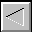|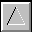|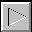|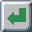|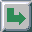TOC -- / --.-- / --.--.-- | Index | Search | Syntax | Help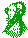-- Email comments, additions, corrections, gripes, kudos, etc. to:

Magnus Kempe -- Magnus.Kempe@di.epfl.ch